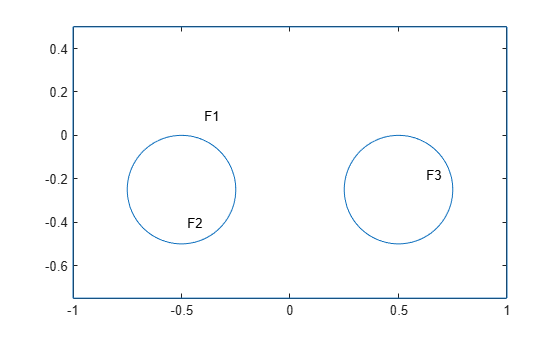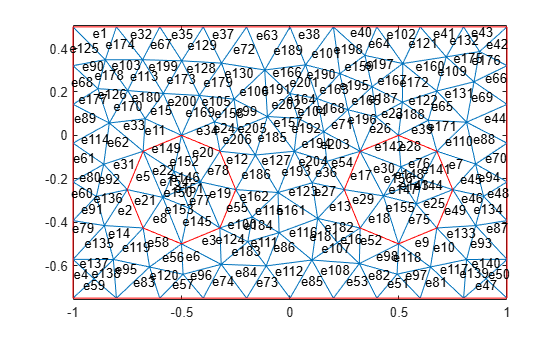# pdesdt

Indices of subset of mesh elements belonging to specified faces of 2-D geometry

`pdesdt` is not recommended. Use `findElements` instead.

## Syntax

``i = pdesdt(t,FaceID)``
``i = pdesdt(t)``

## Description

example

````i = pdesdt(t,FaceID)` returns the indices of the mesh elements of the `[p,e,t]` mesh that belong to the set of faces listed in `FaceID`.```
````i = pdesdt(t)` assumes that `FaceID` is a list of all faces of a 2-D geometry.```

## Examples

collapse all

Find the indices of the mesh elements belonging to the specified faces.

Define two circles and a rectangle and place these in one matrix.

```R1 = [3,4,-1,1,1,-1,0.5,0.5,-0.75,-0.75]'; C1 = [1,-0.5,-0.25,0.25]'; C2 = [1,0.5,-0.25,0.25]'; C1 = [C1;zeros(length(R1) - length(C1),1)]; C2 = [C2;zeros(length(R1) - length(C2),1)]; gd = [R1,C1,C2];```

Create a set formula that adds the circles to the rectangle.

`sf = 'R1+C1+C2';`

Create and plot the geometry.

```ns = char('R1','C1','C2'); ns = ns'; gd = decsg(gd,sf,ns); pdegplot(gd,'FaceLabels','on')```Create a mesh.

`[p,e,t] = initmesh(gd);`

Plot the mesh with the element labels.

`pdemesh(p,e,t,'ElementLabels','on')`Find the indices of the mesh elements that belong to face 2. Display the result as a column vector.

```i = pdesdt(t,2); i.'```
```ans = 16×1 5 8 19 20 21 22 75 76 139 140 ⋮ ```

## Input Arguments

collapse all

Mesh elements, specified as a `4`-by-`Nt` matrix of triangles, where `Nt` is the number of triangles in the mesh. For details on the mesh data representation, see `initmesh`.

Data Types: `double`

Face IDs, specified as a vector of integers.

Data Types: `double`

## Output Arguments

collapse all

Mesh elements belonging to the specified faces, returned as a vector of integers.

### Topics

Introduced before R2006a

## SupportGet trial now